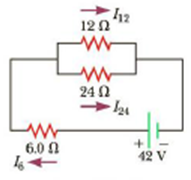Chapter 18, Problem 18P

Chapter
Section
Textbook Problem

(a) Find the current in each resistor of Figure P18.18 by using the rules for resistors in series and parallel. (b) Write three independent equations for the three currents using Kirchhoff’s laws: one with the node rule; a second using the loop rule through the battery, the 6.0-Ω resistor, and the 24.0-Ω resistor; and the third using the loop rule through the 12.0-Ω and 24.0-Ω resistors. Solve to check the answers found in part (a).Figure Pl8.18

(a)

To determine
The current in each resistor from the figure 1.

Explanation

Given Info: The resistance of the parallel combination is R12=12Ω and R24=24Ω , the resistance between the points a and b is R6=6.0Ω , The potential difference between the points a and e is 42V and resistance between the point’s a and e is 14.0Ω .

Explanation:

The following figure shows the current in each resistor.

Formula to calculate the equivalent resistance of the parallel combination between the point’s b and e resistors is,

1Rbe=1R12+1R24

• Rbe is the equivalent resistance between the point’s b and e,
• R12 and R24 is the resistors,

Substitute 12Ω for R12 and 24Ω for R24 .

1Rbe=112Ω+124ΩRbe=24Ω3=8.0Ω

Thus, the equivalent resistance of the parallel combination between the points b and e is 8.0Ω .

Formula to calculate the total resistance between the point’s a and e resistors is,

Rae=Rab+Rbe

• Rae is the total resistance between the point’s a and e,
• Rab is the resistance between the point’s a and b,

Substitute 6.0Ω for Rab and 8.0Ω for Rbe .

Rae=6.0Ω+8.0Ω=14.0Ω

Thus, the total resistance between the point’s a and e is 14.0Ω .

Formula to calculate the total current supplied by the battery is,

Itotal=ΔVaeRae

• Itotal is the total current,
• ΔVae is the potential difference between the points a and e,

Substitute 42V for ΔVae , and 14.0Ω for Rae .

Itotal=42V14

(b)

To determine
The current in each resistor from part (a) and check the answer.

Still sussing out bartleby?

Check out a sample textbook solution.

See a sample solution

The Solution to Your Study Problems

Bartleby provides explanations to thousands of textbook problems written by our experts, many with advanced degrees!

Get Started

Alcohol is a natural substance and therefore does no real damage to body tissues. T F

Nutrition: Concepts and Controversies - Standalone book (MindTap Course List)

In what ways is the celestial sphere a scientific model?

Horizons: Exploring the Universe (MindTap Course List)

Match the terms and definitions.

Human Biology (MindTap Course List)